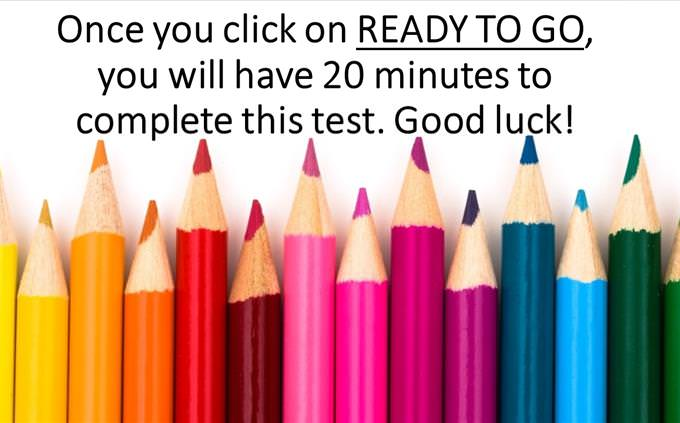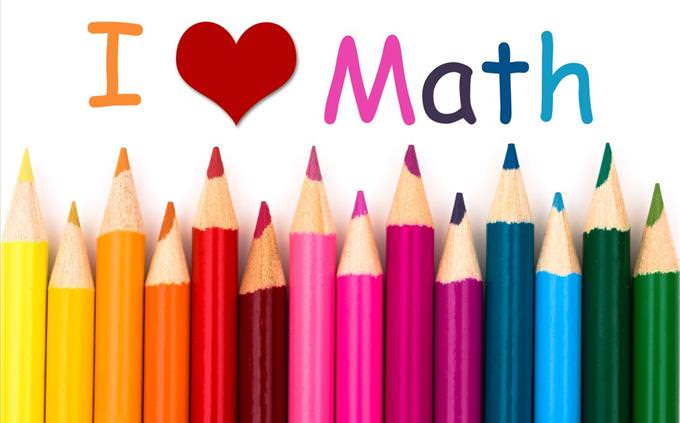# Solve 15 Fun Questions in 20 Minutes!

Welcome to another math quiz! This time we have a really fun quiz for you. Most of the questions are pretty easy, but we've got you on the clock. Don't worry, most of you should have more than enough time to complete these, but keep your eye on it! Another way of saying would be that you have 1200 seconds to complete 15 questions - GO!What is the solution? 3,456 + 721 =
5,007
4,177
3,994What is the solution? 256 - 342 =
-72
-86
-43What is the volume of the cube?
50
125
150What is the solution? 872 ÷ 4 =
143
114
218
244Of the following fractions, which is less than 2/3?
3/5
6/8
4/6Which fraction is equal to 24%?
6/25
4/20
5/25
7/22Two angles in a triangle equal 120°. What is the measure of the third angle?
60°
40°
20°
80°What is the solution? 3(2y + 5) = 3
y = 3
y = 2
y = -2What is the value of C?
13
7
6When you add the square root of 81 to 12 squared, what do you get?
121
21
117
153½ of ½ of ½ of ½ of 64 = ?
2
4
8
1620 ÷ 4 x 10 ÷ 2 = ?
5.5
10
25
18What is the solution? 2 + 1 ÷ 1 + 1 x 2 = ?
2
0
5
32220/20 = ?
109
110
112
111When you add the square root of 100 to 100 squared, what do you get?
10,010
10,110
10,100
10,000